Next: Momentum Representation Up: Position and Momentum Previous: Schrödinger Representation

# Generalized Schrödinger Representation

In the preceding section, we developed the Schrödinger representation for the case of a single operatorcorresponding to a classical Cartesian coordinate. However, this scheme can easily be extended. Consider a system withgeneralized coordinates,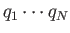, which can all be simultaneously measured. These are represented ascommuting operators,, each with a continuous range of eigenvalues,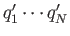. Ket space is conveniently spanned by the simultaneous eigenkets of, which are denoted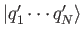. These eigenkets must form a complete set, otherwise thewould not be simultaneously observable.

The orthogonality condition for the eigenkets [i.e., the generalization of Equation (2.26)] is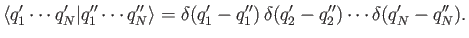(2.59)

The completeness condition [i.e., the generalization of Equation (2.27)] is(2.60)

The standard ket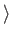is defined such that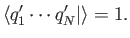(2.61)

The standard bra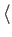is the dual of the standard ket. A general state ket is written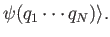(2.62)

The associated wavefunction is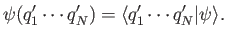(2.63)

Likewise, a general state bra is written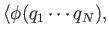(2.64)

where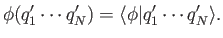(2.65)

The probability of an observation of the system simultaneously finding the first coordinate in the range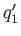to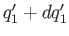, the second coordinate in the range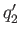to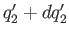, et cetera, is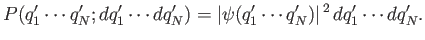(2.66)

Finally, the normalization condition for a physical wavefunction is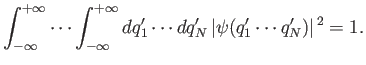(2.67)

Thelinear operators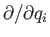(where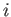runs from 1 to) are defined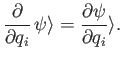(2.68)

These linear operators can also act on bras (provided the associated wavefunctions are square integrable) in accordance with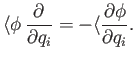(2.69)

[See Equation (2.42).] Corresponding to Equation (2.46), we can derive the commutation relations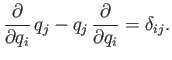(2.70)

It is also clear that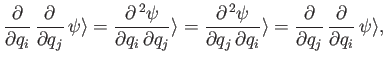(2.71)

showing that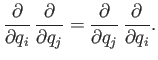(2.72)

It can be seen, by comparison with Equations (2.23)-(2.25), that the linear operators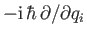satisfy the same commutation relations with the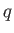's and with each other that the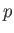's do. The most general conclusion that we can draw from this coincidence of commutation relations is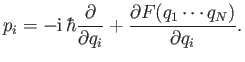(2.73)

However, the function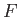can be transformed away via a suitable readjustment of the phases of the basis eigenkets . (See Section 2.4.) Thus, we can always construct a set of simultaneous eigenkets offor which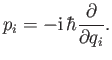(2.74)

This is the generalized Schrödinger representation.

It follows from Equations (2.61), (2.68), and (2.74) that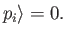(2.75)

Thus, the standard ket in the Schrödinger representation is a simultaneous eigenket of all the momentum operators belonging to the eigenvalue zero. Note that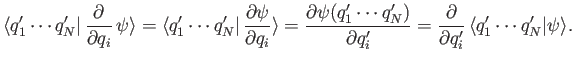(2.76)

Hence,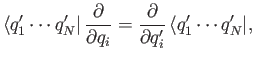(2.77)

so that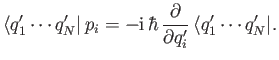(2.78)

The dual of the previous equation gives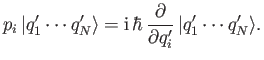(2.79)Next: Momentum Representation Up: Position and Momentum Previous: Schrödinger Representation
Richard Fitzpatrick 2016-01-22